# NCERT Solutions For Class 6 Maths Ratios and Proportions Exercise 12.3

NCERT Solutions For Class 6 Maths Ratios and Proportions Exercise 12.3

### NCERT Solutions For Class 6 Maths Ratios and Proportions Exercise 12.3

NCERT Solutions For Class 6 Maths Chapter 12 Ratios and Proportions Ex 12.3

Exercise 12.3

Ex 12.3 Class 6 Maths Question 1.
If the cost of 7 m of cloth is ₹ 294, find the cost of 5 m of cloth.
Solution:Ex 12.3 Class 6 Maths Question 2.
Ekta earns ₹ 1500 in 10 days. How much she will earn in 30 days?
Solution:Ex 12.3 Class 6 Maths Question 3.
If it has rained 276 mm in the last 3 days, how many centimeters of rain will fall in one full week (7 days)? Assume that the rain continues to fall at the same rate.
Solution:Ex 12.3 Class 6 Maths Question 4.
Cost of 5 kg of wheat is ₹ 30.50.
(a) What will be the cost of 8 kg of wheat?
(b) What quantity of wheat can be purchased in ₹ 61?
Solution:(b) The quantity of wheat purchased in ₹ 30.50 = 5 kgEx 12.3 Class 6 Maths Question 5.
The temperature dropped 15 degree Celsius in the last 30 days. If the rate of temperature drop remains the same, how many degrees will the temperature drop in the next ten days?
Solution:Ex 12.3 Class 6 Maths Question 6.
Shaina pays ₹ 7500 as rent for 3 months. How much does she has to pay for a whole year, if the rent per month remains same?
Solution:Ex 12.3 Class 6 Maths Question 7.
Cost of 4 dozen bananas is ₹ 60. How many bananas can be purchased for ₹ 12.50?
Solution:Ex 12.3 Class 6 Maths Question 8.
The weight of 72 books is 9 kg. What is the weight of 40 such books?
Solution:Ex 12.3 Class 6 Maths Question 9.
A truck requires 108 litres of diesel for covering a distance of 594 km. How much diesel will be required by the truck to cover a distance of 1650 km?
Solution:Ex 12.3 Class 6 Maths Question 10.
Raju purchases 10 pens for ₹150 and Manish buys 7 pens for ₹ 84. Can you say who got the pens cheaper?
Solution:Ex 12.3 Class 6 Maths Question 11.
Anish made 42 runs in 6 overs and Anup made 63 runs in 7 overs. Who made more runs per over?
Solution: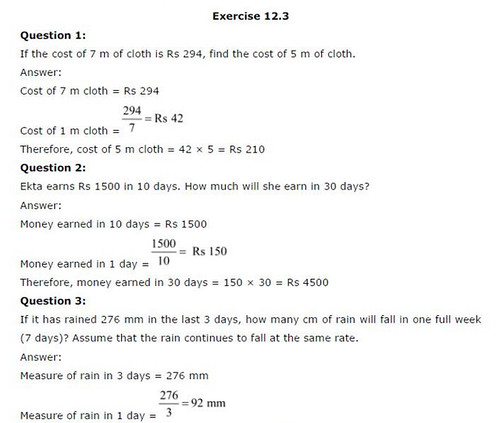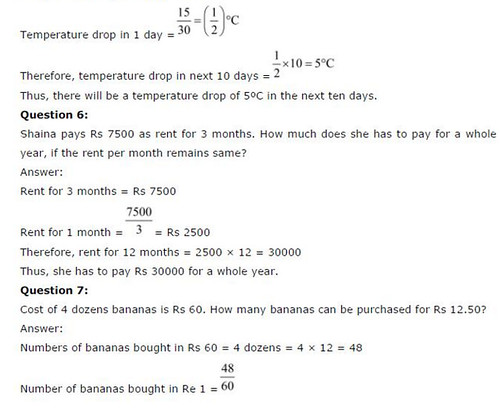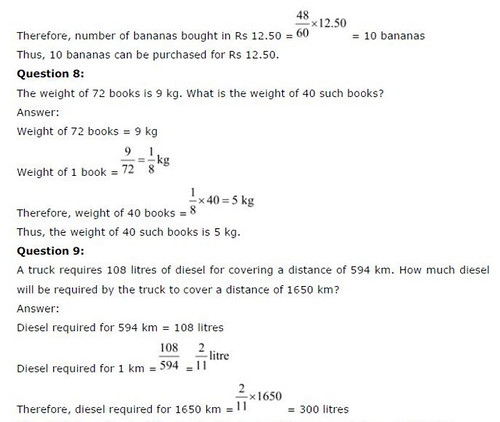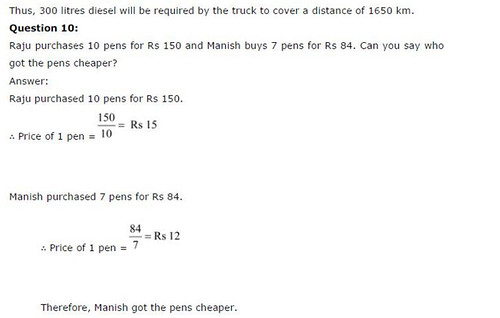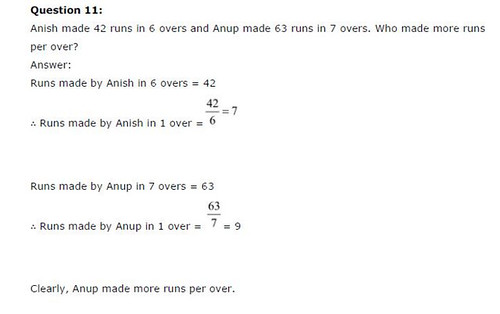## SabDekho

The Complete Educational Website